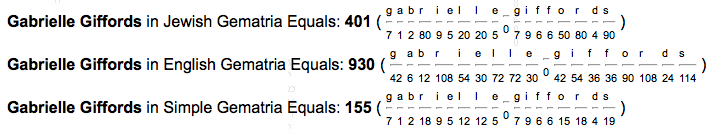## Friday, November 14, 2014

### 33 66 96 113 | 33-Round Magazine Used in Gabrielle Giffords Shooting by Jared Lee Loughner (Hoax Alert)

The Gabby Giffords shooting hoax was the first shooting hoax I covered on this blog.  Back then I overlooked this stat however, the shooter used a 33-round clip... and that he was tackled by a 74-year old man.  I also love the detail that Safeway hasn't released the shooting tape.
• Safeway = 1+1+6+5+5+1+7 = 26
• Safeway sells KJV Bibles
• Savior is written as Saviour in the KJV
• Savior = 1+1+4+9+6+9 = 30
• Saviour = 1+1+4+9+6+3+9 = 33
• Jared = 1+1+9+5+4 = 20
• Lee = 3+5+5 = 13
• Jared Lee = 20+13 = 33
• Loughner = 3+6+3+7+8+5+5+9 = 46
• Jared Loughner = 20+46 = 66
Notice how with Jewish Gematria, his name yield an 113 sequence.  Also, with English Gematria, it sums to 960, yielding the Freemasons' favorite number, 96.

The shooting time of 10:10 is also curious.  A number connected to 'Revelation'.
• Gabrielle = 7+1+2+9+9+5+3+3+5 = 44
• Giffords = 7+9+6+6+6+9+4+1 = 48
The name Giffords also has a connection to Arizona.
• Gabrielle = 7+1+2+18+9+5+12+12+5 = 71 (Seventy-one = 144)
• Giffords = 7+9+6+6+15+18+4+19 = 84 (Arizona = 84) (Obama = 84)